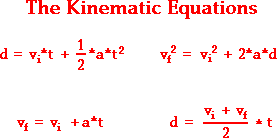# If a projectile is shot at an angle of (5pi)/12 and at a velocity of 15 m/s, when will it reach its maximum height??

Mar 22, 2017

10.711 meters

#### Explanation:

Split the velocity up into x-components and y-components:

$v x = 15 \cos \left(5 \frac{\pi}{12}\right)$

$v y = 15 \sin \left(5 \frac{\pi}{12}\right)$

We only care about the y-component in this case since we want to know maximum height. The initial velocity is $15 \sin \left(5 \frac{\pi}{12}\right)$. The final velocity will be $0$ at the top. The acceleration is always $9.8$ m/s downwards. We need to find d. Find a kinematics equation that has $v i$, $v f$, $a$, and $d$.The equation in the top right corner has all four of those components. Plug into that equation:

$0 = {\left(15 \sin \left(5 \frac{\pi}{12}\right)\right)}^{2} + 2 \left(- 9.8\right) \left(d\right)$

Solve for d:

$d = {\left(- 15 \sin \left(5 \frac{\pi}{12}\right)\right)}^{2} / \left(\left(2\right) \left(- 9.8\right)\right) = 10.711 m$